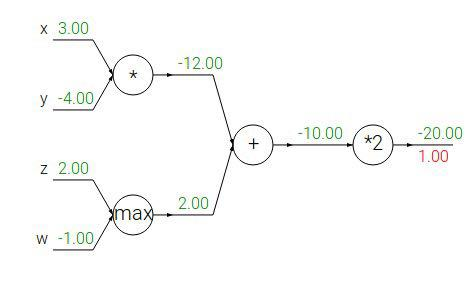DL in NLP 2020. Spring. Quiz 3
Neural Networks. Part 2

Some questions can be not mentioned in the lecture explicitly, but you can still use logic and google.
Credit for some questions: cs231n.stanford.edu
Email address *
Github account *
What is the derivative of a sigmoid function?
How do we compute gradients in backpropagation algorithm?
Default choice of nonlinearity
Why?
What's the main drawback of the ReLU activation function?
y = max(0, x @ W + b), dout - downstream gradient, @ - matrix multiplication, all other operation are element-wise. What is d(loss)/dW?
Gradients with respect to x, y, z, w. Green numbers are forward pass. Red number number is a downstream gradient. Format your answer according to the pattern: x.xx, y.yy, z.zz, w.ww. Example answer: -3.00, 9.60, 18.66, -1.00What is a good way of weights initialization?
Where is the place of the BatchNorm layer in the FFNN architecture?
The BatchNorm layer normalizes data over which axis?
What is a better way of searching neural net's hyperparameters?
Why?
Can we use different learning rates at different layers of a neural network?
When neural network training should be stopped?
What should be done if train loss is much less than validation loss?
Your questions about the lecture (if any)
A copy of your responses will be emailed to the address you provided.
Never submit passwords through Google Forms.
reCAPTCHA
This content is neither created nor endorsed by Google. Report Abuse - Terms of Service - Privacy Policy